# Sinusoidal function

(diff) ← Older revision | Latest revision (diff) | Newer revision → (diff)

## Definition

### As a linear transform of the sine function

The term sinusoidal function refers to a function of the form$f \circ \sin \circ g$ where$f$ and$g$ are linear functions and$\sin$ is the sine function. Specifically, it is a function of the form:$x \mapsto \mu + A\sin(mx + \varphi), \qquad A > 0, m \ne 0$

Here:

•$\mu$ is the mean value about which the function is oscillating, i.e., the graph of a function looks like a scaled sine function about the horizontal line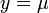$y = \mu$.
•$A$ is the amplitude of oscillations, i.e., the function oscillates between a minimum value of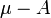$\mu - A$ and a maximum value of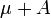$\mu + A$.
•$m$ is the angular frequency parameter and controls the period of oscillations, which is given by$2\pi/m$.
•$\varphi$ is a phase parameter that roughly describes the head start of the function relative to a function that starts at its mean value at$x = 0$.

### As a linear combination of sine and cosine functions

The term sinusoidal function can be used for a function of the form:$x \mapsto \mu + \alpha \sin(mx) + \beta \cos(mx), \qquad m \ne 0, \alpha^2 + \beta^2 > 0$

### Conversion between the two versions

Here's how we convert the linear combination version to the linear transform version:

•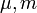$\mu,m$ remain the same.
• Set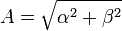$A = \sqrt{\alpha^2 + \beta^2}$.
• Set$\varphi$ as an angle so that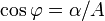$\cos \varphi = \alpha/A$ and$\sin \varphi = \beta/A$.$\varphi$ is uniquely determined up to additive multiples of$2\pi$.

Here's how we convert the linear transform version to the linear combination version:

•$\mu, m$ remain the same.
•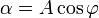$\alpha = A \cos \varphi$.
•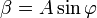$\beta = A \sin \varphi$.

## Examples

Function How it's a sinusoidal function in the linear transform sense How it's a sinusoidal function in the linear combination sense
sine function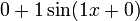$0 + 1\sin(1x + 0)$: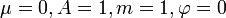$\mu = 0, A = 1, m = 1, \varphi = 0$$0 + 1\sin(1x) + 0\cos(1x)$$\mu = 0, m = 1, \alpha = 1, \beta = 0$
cosine function$0 + 1\sin(1x + \pi/2)$$\mu = 0, A = 1, m = 1, \varphi = \pi/2$$0 + 0\sin(1x) + 1\cos(1x)$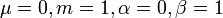$\mu = 0, m = 1, \alpha = 0, \beta = 1$.
sine-squared function$\sin^2$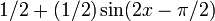$1/2 + (1/2)\sin(2x - \pi/2)$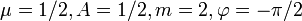$\mu = 1/2, A = 1/2, m = 2, \varphi = -\pi/2$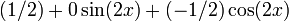$(1/2) + 0\sin(2x) + (-1/2)\cos(2x)$$\mu = 1/2, m = 2, \alpha = 0, \beta = -1/2$
cosine-squared function$\cos^2$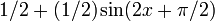$1/2 + (1/2)\sin(2x + \pi/2)$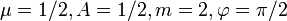$\mu = 1/2, A = 1/2, m = 2, \varphi = \pi/2$$(1/2) + 0\sin(2x) + (1/2)\cos(2x)$$\mu = 1/2, m = 2, \alpha = 0, \beta = 1/2$